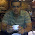## Tuesday, February 5, 2013

### Perl - How to split a string into words?

How to split a string into individual words? Let us see in this article how to split a string which has 2 words separated by spaces.

1. Using the split inbuilt function:
```my \$str="hi  hello";
my (\$var1,\$var2)=split /\s+/,\$str;```
\s+ stands for one or more spaces. By using \s+ as the expression for the split function, it splits everytime it encouters a series of spaces and hence the words are retrieved.

In case multiple words are present in a string, the result can be collected in an array:
```my \$str="hi  hello";
my @arr=split /\s+/,\$str;```
2. Using the regular expression alpha:
```my \$str="hi  hello";
my (\$var1,\$var2)=\$str=~/([[:alpha:]]+)/g;```
[[:alpha:]] will match all the alphabets(lower and upper). So, [[:alpha:]]+ will match a word(hi), and by giving 'g' operator, it keeps find more words and hence "hello" is also retrieved.

3. Using the normal regular expression :
```my \$str="hi  hello";
my (\$var1,\$var2)=\$str=~/([^ ]*)\s+(.*)/;```
This regex matches a set of characters other than space and groups them, and matches a few spaces, and matches the rest of the text which is again grouped. The 1st group contains the 1st word, while the 2nd contains the 2nd word.

4. Using the qr function:
```my \$str="hi  hello";
my \$regex=qr/([^ ]*)\s+(.*)/;
my (\$var1,\$var2)=\$str=~ \$regex;```
qr is a Perl operator used for regular expressions.This operator quotes and compiles the string as a regex. Print the value of the variable \$regex to know how the compiled version of the regular expression looks. Compiled regular expressions are to be preferred when the same regular expression is used in multiple places.1.Can someone help me how to get below output

abc 123
abc 456
abc 789
def 123
def 456
def 789
ghi 123
ghi 456
ghi 789

whereas my input file is
abc 123
456
789
def 123
456
789
ghi 123
456
789

1.perl -ane 'if (@F>1){\$x=\$F;}else{unshift @F,\$x;}print join " ",@F,"\n";' file

2.Can someone help me how to get below output

Input file
------------
123 abc
abc 123

output
---------
123abc
abc123